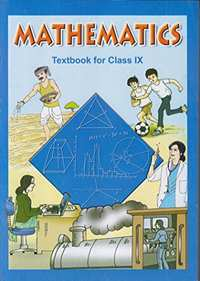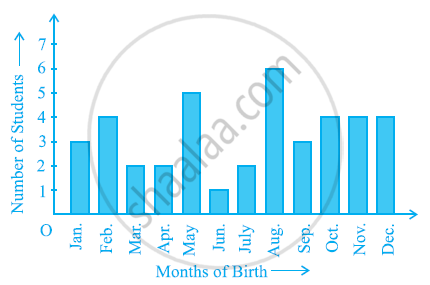# NCERT solutions for Class 9 Maths chapter 15 - Probability [Latest edition]

#### Chapters## Solutions for Chapter 15: Probability

Below listed, you can find solutions for Chapter 15 of CBSE NCERT for Class 9 Maths.

Exercise 15.1
Exercise 15.1 [Pages 284 - 285]

### NCERT solutions for Class 9 Maths Chapter 15 Probability Exercise 15.1 [Pages 284 - 285]

Exercise 15.1 | Q 1 | Page 284

In a cricket match, a batswoman hits a boundary 6 times out of 30 balls she plays. Find the probability that she did not hit a boundary.

Exercise 15.1 | Q 2 | Page 284

1500 families with 2 children were selected randomly, and the following data were recorded:-

 Number of girls in a family 2 1 0 Number of families 475 814 211

Compute the probability of a family, chosen at random, having

(i) 2 girls (ii) 1 girl (iii) No girl

Also check whether the sum of these probabilities is 1.

Exercise 15.1 | Q 3 | Page 284

In a particular section of Class IX, 40 students were asked about the months of their birth and the following graph was prepared for the data so obtained:-Find the probability that a student of the class was born in August.

Exercise 15.1 | Q 4 | Page 284

Three coins are tossed simultaneously 200 times with the following frequencies of different outcomes:-

If the three coins are simultaneously tossed again, compute the probability of 2 heads coming up.

Exercise 15.1 | Q 5 | Page 284

An organization selected 2400 families at random and surveyed them to determine a relationship between income level and the number of vehicles in a family. The information gathered is listed in the table below:-

 Monthly income (in Rs.) Vehicles per family 0 1 2 Above 2 Less than 7000 10 160 25 0 7000 – 10000 0 305 27 2 10000 – 13000 1 535 29 1 13000 – 16000 2 469 59 25 16000 or more 1 579 82 88

Suppose a family is chosen, find the probability that the family chosen is

(i) earning Rs 10000 − 13000 per month and owning exactly 2 vehicles.

(ii) earning Rs 16000 or more per month and owning exactly 1 vehicle.

(iii) earning less than Rs 7000 per month and does not own any vehicle.

(iv) earning Rs 13000 − 16000 per month and owning more than 2 vehicles.

(v) owning not more than 1 vehicle.

Exercise 15.1 | Q 6 | Page 284

A teacher wanted to analyse the performance of two sections of students in a mathematics test of 100 marks. Looking at their performances, she found that a few students got under 20 marks and a few got 70 marks or above. So she decided to group them into intervals of varying sizes as follows: 0 − 20, 20 − 30… 60 − 70, 70 − 100. Then she formed the following table:-

 Marks Number of students 0 - 20 7 20 - 30 10 30 - 40 10 40 - 50 20 50 - 60 20 60 - 70 15 70 - above 8 Total                               90

(i) Find the probability that a student obtained less than 20 % in the mathematics test.

(ii) Find the probability that a student obtained marks 60 or above.

Exercise 15.1 | Q 7 | Page 284

To know the opinion of the students about the subject statistics, a survey of 200 students was conducted. The data is recorded in the following table.

 Opinion Number of students like 135 dislike 65

Find the probability that a student chosen at random

(i) likes statistics, (ii) does not like it

Exercise 15.1 | Q 8 | Page 284

The distance (in km) of 40 engineers from their residence to their place of work were found as follows.

 5 3 10 20 25 11 13 7 12 31 19 10 12 17 18 11 32 17 16 2 7 9 7 8 3 5 12 15 18 3 12 14 2 9 6 15 15 7 6 12

What is the empirical probability that an engineer lives:-

(i) less than 7 km from her place of work?What is the empirical probability that an engineer lives:

(ii) more than or equal to 7 km from her place of work?

(iii) within 1/2 km from her place of work?

Exercise 15.1 | Q 11 | Page 285

Eleven bags of wheat flour, each marked 5 kg, actually contained the following weights of flour (in kg):- 4.97, 5.05, 5.08, 5.03, 5.00, 5.06, 5.08, 4.98, 5.04, 5.07, 5.00

Find the probability that any of these bags chosen at random contains more than 5 kg of flour.

Exercise 15.1 | Q 12 | Page 285
 Concentration of SO2 (in ppm) Number of days (Frequency) 0.00 − 0.04 4 0.04 − 0.08 9 0.08 − 0.12 9 0.12 − 0.16 2 0.16 − 0.20 4 0.20 − 0.24 2 Total 30

The above frequency distribution table represents the concentration of sulphur dioxide in the air in parts per million of a certain city for 30 days. Using this table, find the probability of the concentration of sulphur dioxide in the interval 0.12 − 0.16 on any of these days.

Exercise 15.1 | Q 13 | Page 285
 Blood Group Number of Students A 9 B 6 AB 3 O 12 Total 30

The above frequency distribution table represents the blood groups of 30 students of a class. Use this table to determine the probability that a student of this class, selected at random, has blood group AB.

## Solutions for Chapter 15: Probability

Exercise 15.1## NCERT solutions for Class 9 Maths chapter 15 - Probability

Shaalaa.com has the CBSE Mathematics Class 9 Maths CBSE solutions in a manner that help students grasp basic concepts better and faster. The detailed, step-by-step solutions will help you understand the concepts better and clarify any confusion. NCERT solutions for Mathematics Class 9 Maths CBSE 15 (Probability) include all questions with answers and detailed explanations. This will clear students' doubts about questions and improve their application skills while preparing for board exams.

Further, we at Shaalaa.com provide such solutions so students can prepare for written exams. NCERT textbook solutions can be a core help for self-study and provide excellent self-help guidance for students.

Concepts covered in Class 9 Maths chapter 15 Probability are Probability - an Experimental Approach.

Using NCERT Class 9 Maths solutions Probability exercise by students is an easy way to prepare for the exams, as they involve solutions arranged chapter-wise and also page-wise. The questions involved in NCERT Solutions are essential questions that can be asked in the final exam. Maximum CBSE Class 9 Maths students prefer NCERT Textbook Solutions to score more in exams.

Get the free view of Chapter 15, Probability Class 9 Maths additional questions for Mathematics Class 9 Maths CBSE, and you can use Shaalaa.com to keep it handy for your exam preparation.

Share